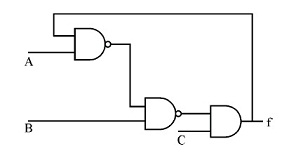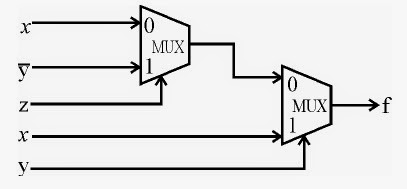81. Consider the circuit shown below. In a certain steady state, Y is at logical ‘l’. What are possible values of A, B, C ?a. A = 0, B = 0, C = 1 b. A = 0, B = C = 1 c. A = B = 1, C = 1 d. either A or C
 Answer: (d).either A or C

 82. The branch logic that provides making capabilities in the control unit is known as a. Controlled transfer b. Conditional transfer c. Unconditional transfer d. None of the above

 83. Cached and interleaved memories are ways of speeding up memory access between CPU’s and slower RAM. Which memory models are best suited (i.e. improves the performance most) for which programs ?(i) Cached memory is best suited for small loops.(ii) Interleaved memory is best suited for small loops(iii) Interleaved memory is best suited for large sequential code.(iv) Cached memory is best suited for large sequential code. a. (i) and (ii) are true b. (i) and (iii) are true c. (iv) and (ii) are true d. (iv) and (iii) are true
 Answer: (b).(i) and (iii) are true

 84. Which of the following logic families is well suited for high-speed operations ? a. TTL b. ECL c. MOS d. CMOS

 85. A complete microcomputer system consists of a. Microprocessor b. Memory c. Peripheral equipment d. All of the above
 Answer: (d).All of the above

 86. Where does a computer add and compare data ? a. Hard disk b. Floppy disk c. CPU chip d. Memory chip

 87. Pipelining strategy is called implement a. instruction execution b. instruction prefetch c. instruction decoding d. instruction manipulation
 90. Consider the below circuit and find the output function f(x,y,z).a. xz’+xy+y’z b. xz’+xy+y’z’ c. xz+xy+y’z’ d. xz+xy’+y’z